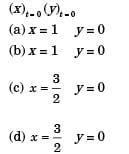Consider a rotating disk cam and a translating roller follower with zero offset. Which one of the following pitch curves, parameterized by t, lying in the interval 0 to 2π, is associated with the maximum translation of the follower during one full rotation of the cam rotating about the center at (x, y) = (0, 0)?

• a)
x(t) = cost, y(t) = sint
• b)
x(t) = cost, y(t) = 2 sint
• c)
x(t) =(1/2) + cost, y(t) = 2sint
• d)
x(t) =(1/2) + cost, y(t) = sint

### Related TestManish Aggarwal Mar 31, 2021